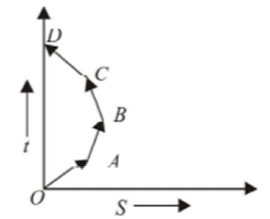Q.1
##### Which of the following option is correct for the object having a straight line motion represented by the following graph• average velocity is zero
• velocity of the object increases uniformly
• the object moves with constantly increasing velocity from O to A and then it moves with constant velocity
• the graph shown is impossible
Q.2
• 110 m/s
• 550 m/s
• 650 m/s
• 55 m/s
Q.3
• 4 m/s
• 5 m/s
• 5.5 m/s
• 4.8 m/s
Q.4
##### A particle moving along x-axis has acceleration f at time t, given by $f=f_0 \frac {t}{T}$,where f_0 and T are constants. The particle at t=0 has zero velocity. In the time interval between t=0 and the instant when f=0, the particle’s velocity $v_x$ is
• $\frac {1}{2} f_0 T^2$
• $f_0 T^2$
• $\frac {1}{2} f_0 T$
• $f_0 T$
Q.5
##### The relation between time t and distance x is $t=ax^2+bx$,where a and b are constants. The acceleration is
• $-2abv^2$
• $2bv^3$
• $-2av^3$
• $2av^2$
Q.6
• 40 m/s
• 26.1 m/s
• 9.6 m/s
• 55.5 m/s
Q.7
• 12 m
• 18 m
• 6 m
• 24 m
Q.8
##### Two boys are standing at the ends A and B of a ground, where AB=a. The boy at B starts running in a direction perpendicular to AB with velocity  The boy at A starts running simultaneously with velocity v and catches the other by in a time t, where t is
• $\frac {a}{\sqrt {v^2+v_1^2 }}$
• $\sqrt { \frac {a^2}{v^2 -v_1^2}}$
• $\frac {a}{v-v_1}$
• $\frac {a}{v+v_1}$
Q.9
• 4 sec
• 8 sec
• 16 sec
• 10 sec
Q.10
• 12 m/s
• 36 m/s
• 27 m/s
• 18 m/s
Q.11
• a
• b
• c
• d